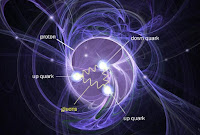## QuestionPoint particles in proton

For a system of discrete point particles the energy-momentum tensor takes the form$$T_{\mu\nu}=\sum_{a}{\frac{p_\mu^{\left(a\right)}p_\nu^{\left(a\right)}}{p^{0\left(a\right)}}\delta^{\left(3\right)}\left(\mathbf{x}-\mathbf{x}^{\left(a\right)}\right)}$$where the index $a$ labels the different particles. Show that, for a dense collection of particles with isotropically distributed velocities, we can smooth over the individual particle worldlines to obtain the perfect-fluid energy-momentum tensor 1.114.

1.114 was$$\mathbf{T}^{\mu\nu}=\left(\rho+p\right)U^\mu U^\nu+p\eta^{\mu\nu}$$where $\rho$ is the energy density of the fluid measured in its rest frame (rest-frame energy density) and $p$ is the isotropic rest-frame pressure. $T^{\mu\nu}$ is the energy-momentum tensor of a fluid element with a four velocity $U^\mu$.

• The $p$'s in second equation are rest-frame pressure and the $p$'s in the first are 4-momentum components.
• What does $\delta^{\left(3\right)}\left(\mathbf{x}-\mathbf{x}^{\left(a\right)}\right)$ mean? Carroll gives no hint.
First I found out what $\delta^{\left(3\right)}\left(\mathbf{x}-\mathbf{x}^{\left(a\right)}\right)$ is and justified the expression for the energy-momentum of the point particle. I then got stuck until I found a solution by Alexey Bobrick on physics.stackexchange. It starts well but ends not so well, I believe. I think I did a little better.